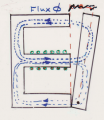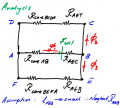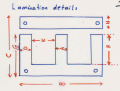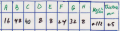# Optimizing the design of a magnetic actuator

#### TheRedDevil18

Joined May 23, 2015
14
Hi,I have a project where I have to try and get the maximum force/weight ratio for a magnetic actuator within a set of constraints. I am working on the calculations for the force but I need to know if I am on the right track so I need some help

Actuator:Equivalent Circuit:Lamination details:Dimensions:These are the constraints:
12V dc input supply
Current not to exceed 1A in the coil
Maximum depth of core is 16 mm
Lamination type, hot rolled silicon steel

Parameters under my control:
Number of turns on coil
Area of the core
Top air gap width

All dimensions are in mm. The 0.5mm is the thickness per lamination.

I will show some of my work here what iv'e done so far:

The first thing is to calculate the current in the coil:

Using, R = p*length of wire/Area of wire , p = resistivity of copper wire

Length wire = Number of turns*Perimeter of bobbin , Perimeter of bobbin = 74mm

Area of wire = (pi*d^2)/4, where d is diameter of wire = 0.32 mm

I am thinking I should be aiming for maximum current in the coil to get maximum mmf ?
So I chose as a start:
Number of turns = 775
Using V = IR, where V = 12V (fixed)
I = 0.97 A

So, Total mmf = NI
= 775*0.97
= 751.75 At

For the air gap, I am thinking I should make it small so as to get a higher H value ?
So I chose as a start:

Top air gap = 0.5 mm
Using similar triangles:
lgt/40 = lgc/20 where lgt = top air gap (0.5mm) and lgc = centre air gap
lgc = 0.25mm

I can now calculate the air gap reluctances, as follows:
Rgt = Lgt/u*Agt
Rgc = Lgc/u*Agc

where Rgt and Rgc are the top and centre air gap reluctances

For the area, i'm not too sure. If I add too many laminations the mass will increase
So I chose as a start:

16 laminations*0.5mm thickness = 8mm depth

So, Agt = 8*8 = 64 mm^2
Agc = 16*8 = 128 mm^2

Substituting I get:
Rgc = 1.55*10^6 At/Wb
Rgt = 6.22*10^6 At/Wb

The next part I need to estimate the mmf drops in the air gap, so I estimated 90% drop in the combined Rgt and Rgc. This part I am not sure off but I know that mmf will be greatest in the air gap

So, 0.9*751.75 (Total mmf) = 676.575 At for Rgt and Rgc

I then divided that mmf individually between Rgt and Rgc using the ratio:
20:80 , ie 20% for Rgc and 80% for Rgt. I am also not too sure about this part

So, 0.2*676.575 = 135.315 = mmf of Rgc (Fgc)
and 0.8*676.575 = 541.26 = mmf of Rgt (Fgt)

Now, I found B using the formula
Bgc = Fgc*u/lgc
Bgt = Fgt*u/lgt

Substituting I get Bgc = 0.68 T = magnetic field of RcoreAB because area and flux is same

and, Bgt = 1.36 T = magnetic field of RcoreBCDA because area and flux is same

Using the BH curve for hot rolled silicon steel, I get
Hgt = 660 At/m
Hgc = 40 At/m

Now using the formula F = HL, I can find the remaining mmf core drops

I hope that wasn't too long, can you please see if i did it correctly, thanks

#### Alec_t

Joined Sep 17, 2013
12,311
Welcome to AAC!
I haven't checked all your maths, but here are some things to consider:-
The flux is proportional to both the current and the number of turns.
Be aware of core flux saturation.
Do you have space on your bobbin to accomodate the number of turns you want using the wire gauge available/necessary?
Have you thought about heat dissipation?

#### TheRedDevil18

Joined May 23, 2015
14
Welcome to AAC!
I haven't checked all your maths, but here are some things to consider:-
The flux is proportional to both the current and the number of turns.
Be aware of core flux saturation.
Do you have space on your bobbin to accomodate the number of turns you want using the wire gauge available/necessary?
Have you thought about heat dissipation?
Thanks for replying. Due to this being a very basic project we are not required to worry about heat dissipation, and yes I do have space on the bobbin

I have a question, I'm trying to work out the fluxes and mmf drops. Can I use KVL for each loop, but instead calculate mmf drops ?

#### Alec_t

Joined Sep 17, 2013
12,311
I'm not well up on magnetics, but using a magnetic analogue of an electrical method seems reasonable, e.g. emf/mmf, resistance/reluctance ...

#### TheRedDevil18

Joined May 23, 2015
14
Okay, I tried KVL and this is what I got

This is my KVL equation:
Top loop:

Ftotal = (phi1*RcoreAB) + (phi1*Rgc) + (phi2*Rgt) + (phi2*RcoreBCDA)

Bottom loop:

Ftotal = (phi1*RcoreAB) + (phi1*Rgc) + (phi3*RcoreBEFA)

If I simplify by equating them and saying that RcoreBEFA = RcoreBCDA, I get this expression

phi3/phi2 = (Rgt/RcoreBEFA) + 1

I'm not sure if that's right ?, also will RcoreBCDA = RcoreBEFA ?, thanks